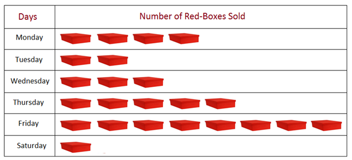Mathematics
Easy

Question

# How many red boxes were sold on Friday?## 10358Hint:

## The correct answer is: 8

###Here we have to find the number of red boxes were sold on Friday.Firstly, In fig.., we given by each days and also the number of red boxes sold,On Friday , see on table then,Count the total number of boxes,Therefore, the total number of red boxes were sold on Friday is 8..The correct answer is 8 (option (d)).

In this question , We have given by table we just find out the given day and count the number of boxes in the row.

### Related Questions to study#### With Turito Foundation.#### Get an Expert Advice From Turito.